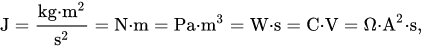# How to convert joules to volts

How to convert energy in joules (J) to electrical voltage in volts (V).

You can calculate volts from joules and coulombs, but you can't convert joules to volts since volt and joule units represent different quantities.

### Joules to volts calculation formula

The voltage V in volts (V) is equal to the energy E in joules (J), divided by the charge Q in coulombs (C):

V(V) = E(J) / Q(C)

So

volt = joule / coulomb

or

V = J / C

#### Example

What is the voltage supply of an electrical circuit with energy consumption of 60 joules and charge flow of 4 coulombs?

V = 60J / 4C = 15V

### Volt definition

Volt is the electrical unit of voltage or potential difference (symbol: V).

One Volt is defined as energy consumption of one joule per electric charge of one coulomb.

1V = 1J/C

One volt is equal to current of 1 amp times resistance of 1 ohm:

1V = 1A ⋅ 1Ω

### Joule definition

The joule is a derived unit of energy in the International System of Units. It is equal to the energy transferred to (or work done on) an object when a force of one newton acts on that object in the direction of the force's motion through a distance of one metre (1 newton metre or N⋅m). It is also the energy dissipated as heat when an electric current of one ampere passes through a resistance of one ohm for one second. It is named after the English physicist James Prescott Joule (1818–1889).One joule can also be defined as the following:

• The work required to move an electric charge of one coulomb through an electrical potential difference of one volt, or one coulomb-volt (C⋅V). This relationship can be used to define the volt.
• The work required to produce one watt of power for one second, or one watt-second (W⋅s) (compare kilowatt-hour – 3.6 megajoules). This relationship can be used to define the watt.

The joule is named after James Prescott Joule. As with every SI unit named for a person, its symbol starts with an upper case letter (J), but when written in full it follows the rules for capitalisation of a common noun; i.e., "joule" becomes capitalised at the beginning of a sentence and in titles, but is otherwise in lower case.

How to convert volts to joules »

Currently, we have around 945 calculators, conversion tables and usefull online tools and software features for students, teaching and teachers, designers and simply for everyone.